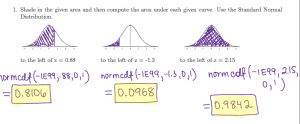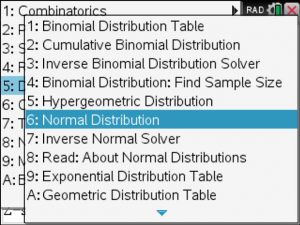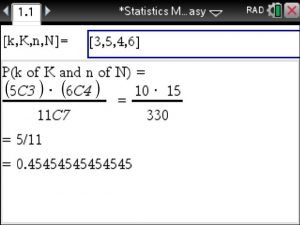## Uniform and Discrete Distribution using the TiNspire CX

Given a Discrete Probability Distribution such as the one shown below make sure that the sum of the given probabilities is 100% or 1 . If you own a TiNspire CX you can enter the distribution in the top two boxes of the Statistics Made Easy App available at www.TiNspireApps.com .

Once done you get the expected value , variance : step by step.
Optional is the value of N which allows to compute P(X>N) , P(X<N) etc

For a uniform distribution , you enter the given interval [a,b] and the value c which lies on [a,b] . You will then find P(X<c) and P(X>c)

Posted on Categories discrete math, distribution, statistics

## NormalDistribution using the TiNspire and Statistics Made Easy

Normaldistributions can easily be dealt with using the TiNspire and the Statistics Made Easy

App from www.TiNspireapps.com.

If you are to compute the area under the Standard Normalcurve as shown in the problems in the image below:Just navigate to Normaldistribution in the Menu :Now enter mean = 1 and std deviation = 0   as we are dealing with the StandardNormal Distribution.

Next enter B as shown given in problem .88 and A can be any number below -3 … out comes the answer

Alternatively, you could have entered .88 for A and found the answer in the 3. line instead of the 2. lineAdditionally, InvNorm type problems (given area find z value ) can also be solved

using this Statistics Made Easy app at www.TiNspireApps.com

## Hypergeometric Distribution on the TiNspire CX CAS in Statistics Made Easy – Step by Step.

Using the Hypergeometric Distribution on the TiNspire CX CAS in Statistics Made Easy at www.TiNspireApps.com

Here is an example:Posted on Categories distribution, hypergeometric, tinspirecx